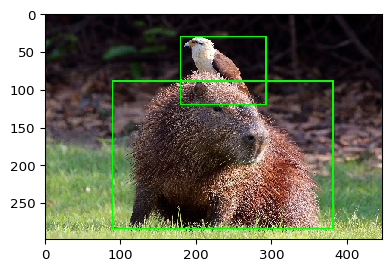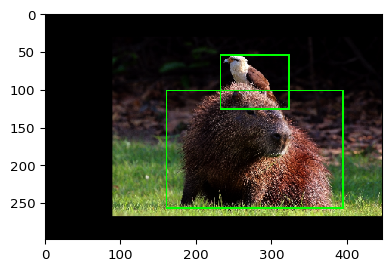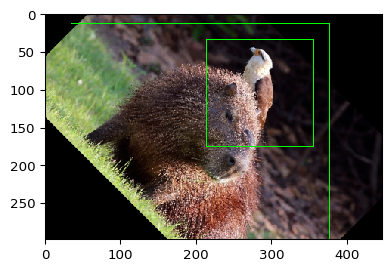# Augment Bounding Boxes¶

imgaug has native support for bounding boxes and their augmentation. They are represented via their top-left and bottom-right corner coordinates, both as absolute values and with sub-pixel accuracy.

In imgaug, bounding boxes are only affected by augmenters changing the geometry of images. This is the case for e.g. horizontal flips or affine transformations. They are not affected by other methods, such as gaussian noise.

Two classes are provided for bounding box augmentation in imgaug, listed in the following sections.

## API: BoundingBox¶

imgaug.augmentables.bbs.BoundingBox(x1, y1, x2, y2, label=None): Container for a single bounding box, defined based on its top-left and bottom-right corners, each given as x- and y- pixel coordinates (floats, i.e. sub-pixel accurate).

• Important properties offered by BoundingBox are: .x1, .y1, .x2, .y2, .height, .width, .area, .center_x, .center_y.
• Important methods offered by BoundingBox are:
• project(from_shape, to_shape): Projects the bounding box coordinates from one image shape to another.
• extend([all_sides], [top], [right], [bottom], [left]): Increases the size of the bounding boxes by pixel values along given sides.
• intersection(other, [default]): Returns the intersection bounding box of this bounding box and another one.
• union(other): Analogous to intersection(), returns the union.
• iou(other): Computes the IoU of this bounding box and another one.
• is_fully_within_image(image): Determines whether all bounding box coordinates are inside the image plane.
• is_partly_within_image(image): Determines whether at least parts of the bounding box are inside the image plane.
• clip_out_of_image(image): Clips off all parts of the bounding box that are outside of the image plane.
• shift([x], [y]): Moves the bounding box.
• draw_on_image(image, [color], [alpha], [size], [copy], [raise_if_out_of_image]): Draws the bounding box and its label on an image.
• draw_label_on_image(image, [color], [color_text], [color_bg], [alpha], [size], [size_text], [height], [copy], [raise_if_out_of_image]): Draws only a rectangle containing the label (text) on an image (above the bounding box).
• draw_box_on_image(image, [color], [alpha], [size], [copy], [raise_if_out_of_image): Draws only the box of the bounding box on an image.
• extract_from_image(image, [pad], [pad_max], [prevent_zero_size]): Extracts the pixels contained in a bounding box from an image.

## API: BoundingBoxesOnImage¶

imgaug.augmentables.bbs.BoundingBoxesOnImage(bounding_boxes, shape): Container for a list of bounding boxes placed on an image. The shape argument denotes the shape of the image on which the bounding boxes are placed. It is required to make sure that augmentations based on the image size are aligned between the image and the bounding boxes placed on it (e.g. cropping).

• Important methods offered by BoundingBoxesOnImage are:
• on(image): Projects the bounding box(es) onto another image.
• from_xyxy_array(xyxy, shape): Creates a BoundingBoxesOnImage instance from an (N,4) numpy array.
• to_xyxy_array([dtype]): Converts the instance to an (N,4) numpy array.
• draw_on_image([color], [alpha], [size], [copy], [raise_if_out_of_image]): Draws all bounding boxes and their labels onto an image.
• remove_out_of_image([fully], [partly]): Removes bounding box that are fully or at least partially outside of the image plane.
• clip_out_of_image(): Calls clip_out_of_image() on all bounding boxes.
• shift([x], [y]): Calls shift() on all bounding boxes.

## API: Methods¶

Bounding boxes can be augmented using the method augment(images=..., bounding_boxes=...). Alternatively, augment_bounding_boxes() can be used, which accepts either a single instance of BoundingBoxesOnImage or a list of that class.

## API: More¶

Most of the mentioned methods are explained below. The API also contains further details. See e.g. BoundingBox, BoundingBoxesOnImage, imgaug.augmenters.meta.Augmenter.augment() and imgaug.augmenters.meta.Augmenter.augment_bounding_boxes().

## Example¶

Let's try a simple example for bounding box augmentation. We load one image, place two bounding boxes on it and then augment the data using an affine transformation.

First, we load and visualize the data:

In :
import imageio
import imgaug as ia
from imgaug.augmentables.bbs import BoundingBox, BoundingBoxesOnImage
%matplotlib inline
ia.seed(1)

image = ia.imresize_single_image(image, (298, 447))

bbs = BoundingBoxesOnImage([
BoundingBox(x1=0.2*447, x2=0.85*447, y1=0.3*298, y2=0.95*298),
BoundingBox(x1=0.4*447, x2=0.65*447, y1=0.1*298, y2=0.4*298)
], shape=image.shape)

ia.imshow(bbs.draw_on_image(image, size=2))The next step is to define the augmentations that we want to apply. We choose a simple contrast augmentation (affects only the image) and an affine transformation (affects image and bounding boxes).

In :
from imgaug import augmenters as iaa
ia.seed(1)

seq = iaa.Sequential([
iaa.GammaContrast(1.5),
iaa.Affine(translate_percent={"x": 0.1}, scale=0.8)
])


Now we augment both the image and the bounding boxes on it. We can use seq.augment(...) for that or its shortcut seq(...):

In :
image_aug, bbs_aug = seq(image=image, bounding_boxes=bbs)


Note that if we wanted to augment several images, we would have used something like seq(images=[image1, image2, ...], bounding_boxes=[bbs1, bbs2, ...]). The method is fairly flexible and can also handle bounding boxes that differ from BoundingBoxesOnImage, e.g. a (N,4) array per image denoting (x1,y1,x2,y2) of each bounding box. Make sure though to call the method once for both images and bounding boxes, not twice (once for images, once for bounding boxes) as then different random values would be sampled per call and the augmentations would end being unaligned.

Now that we have our data augmented we can visualize it again:

In :
ia.imshow(bbs_aug.draw_on_image(image_aug, size=2))## Problems Introduced by Rotation of 45°¶

Let's try a different augmentation technique. This time we apply an affine transformation consisting only of rotation.

In :
image_aug, bbs_aug = iaa.Affine(rotate=45)(image=image, bounding_boxes=bbs)
ia.imshow(bbs_aug.draw_on_image(image_aug))You may now be inclined to say that these augmentations look off and that something must have went wrong. But the outputs are actually correct and show a corner case of bounding box augmentation -- or, why you should avoid 45° rotations. The problem originates from non-object pixels being part of the bounding box. After rotation, a new bounding box has to be drawn that incorporates these non-object pixels. The following example visualizes the problem:

In :
import numpy as np
import matplotlib.pyplot as plt

# highlight the area of each bounding box
image_points = np.copy(image)
colors = [(0, 255, 0), (128, 128, 255)]
for bb, color in zip(bbs.bounding_boxes, colors):
image_points[bb.y1_int:bb.y2_int:4, bb.x1_int:bb.x2_int:4] = color

# rotate the image with the highlighted bounding box areas
rot = iaa.Affine(rotate=45)
image_points_aug, bbs_aug = rot(image=image_points, bounding_boxes=bbs)

# visualize
side_by_side = np.hstack([
bbs.draw_on_image(image_points, size=2),
bbs_aug.draw_on_image(image_points_aug, size=2)
])
fig, ax = plt.subplots(figsize=(20, 20))
ax.imshow(side_by_side)

Out:
<matplotlib.image.AxesImage at 0x7f30e42480f0>